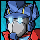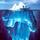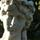General QuestionWhat mathematical sequence includes these two numbers?

Asked by weeveeship (4660) December 15th, 2010

The numbers are:

130812782.6503 (28th number in the sequence)
523251130.6012 (52nd number in the sequence)

P.S. I think the sequence is mathematical because it involves numbers but feel free to go beyond math sequences. Thanks a bunch.

Observing members: 0Composing members: 0The ratio of 52nd to 28th term is exactly 4. This is the only real clue here.

This suggests a geometric series, in which case the terms quadruple every 52 – 28 = 24 positions, or double every 12. This is exactly the case with the well-tempered piano scale, where frequencies double each octave of 12 semi-tones. The ratio between successive terms is the twelfth root of two, which is 2^(1/12) = 1.059… (Each note is about 6 percent higher in frequency than its predecessor).

What puzzles me is that the numbers in your sequence are on the order of a million times bigger than piano frequencies in cycles per second (Hertz).

gasman (11315)“Great Answer” (12) Flag as…@gasman

Having frequencies double every 12 semi-tones is not unique to well temperament. The feature of well temperament is the relative frequencies within the octave. I’m quite sure nearly all Western tuning methods have a 2:1 frequency ratio every 12 semi-tones, otherwise you would not be able to sustain a scale over a wide range of notes.

chocolatechip (3004)“Great Answer” (1) Flag as…@chocolatechip I understand—forgive me for not explaining it well. I meant that in the context of geometric / exponential series, where the ratio of each term to its predecessor is constant, it seems more than a coincidence to have exact doubling after exactly 12 steps.

Earlier natural musical scales (where a perfect fifth is a 3:2 frequency ratio, etc.) still feature octave doubling, as you point out, but then the tones do not form a perfectly geometric series. My answer was based on assuming a geometric series. This is more or less a leap of faith, given practically no other information to work with.

gasman (11315)“Great Answer” (1) Flag as…or